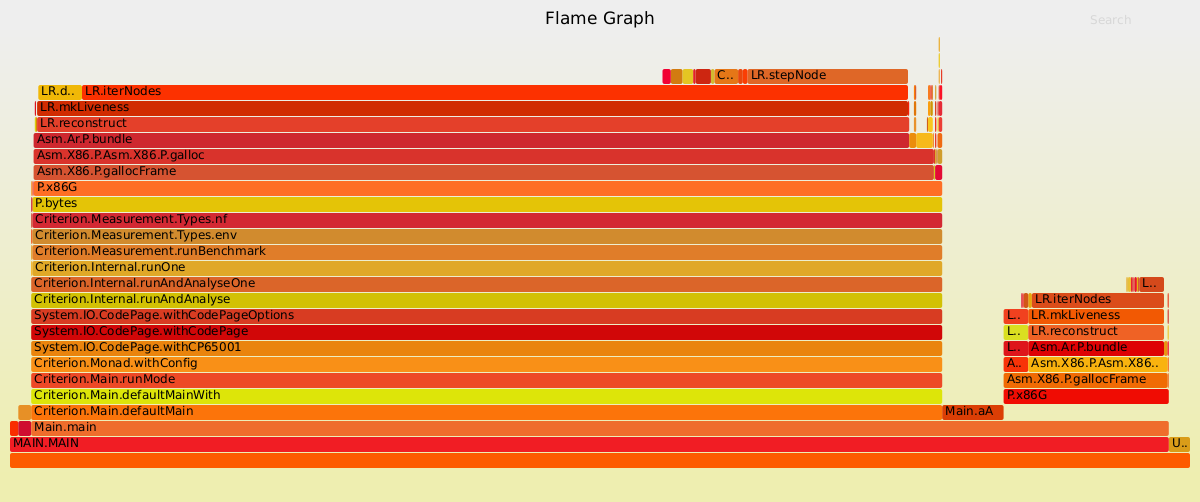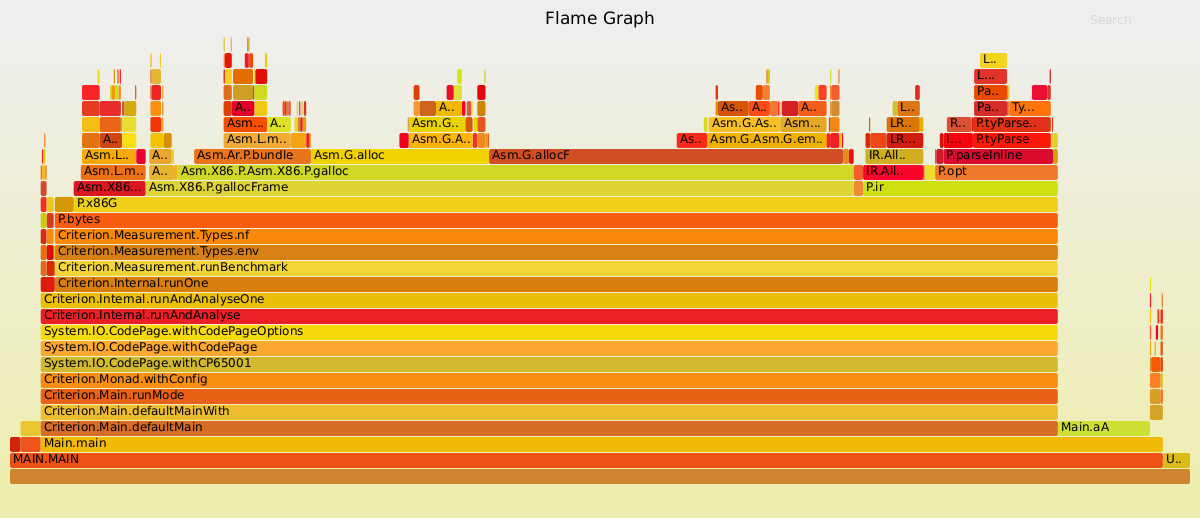Building a respectable compiler requires basic blocks in order for liveness analysis to be performant. Consider my own Apple compiler:Compilation was dominated by mkLiveness and moreover simple functions took tens of milliseconds to compile.

benchmarking pipeline/bytes (gamma)
time                 62.52 ms   (62.14 ms .. 62.86 ms)
1.000 R²   (1.000 R² .. 1.000 R²)
mean                 62.54 ms   (62.29 ms .. 62.80 ms)
std dev              470.3 μs   (308.3 μs .. 657.9 μs)benchmarking pipeline/bytes (fcdf)
time                 108.3 ms   (106.6 ms .. 110.5 ms)
1.000 R²   (0.999 R² .. 1.000 R²)
mean                 106.1 ms   (105.4 ms .. 107.1 ms)
std dev              1.288 ms   (696.6 μs .. 2.067 ms)

After rewriting to use basic blocks, compilation times were less than 20ms, a far better user experience. Moreover, liveness analysis no longer dominates.

benchmarking pipeline/bytes (gamma)
time                 5.313 ms   (5.171 ms .. 5.485 ms)
0.994 R²   (0.991 R² .. 0.997 R²)
mean                 5.166 ms   (5.087 ms .. 5.262 ms)
std dev              267.0 μs   (218.5 μs .. 321.2 μs)
variance introduced by outliers: 29% (moderately inflated)benchmarking pipeline/bytes (fcdf)
time                 10.42 ms   (9.838 ms .. 10.96 ms)
0.982 R²   (0.971 R² .. 0.991 R²)
mean                 12.13 ms   (11.71 ms .. 12.55 ms)
std dev              1.090 ms   (907.0 μs .. 1.409 ms)
variance introduced by outliers: 48% (moderately inflated)Appel mentions basic blocks as an advanced optimization but does not fill in details; the precise implementation in Haskell is instructive and elegant.

First, we define uBB, dBB to simulate uses and defs on a basic block:

uBB, dBB :: E reg => [X86 reg freg a] -> IS.IntSet
uBB = foldr (\p n -> uses p IS.union (n IS.\\ defs p)) IS.empty
dBB = foldMap defs

Note that foldr processes instructions in reverse execution order.

Then, to get liveness information for each instruction, we apply the liveness equations (each instruction has one successor in a basic block):

$${\tt in}[n]={\tt uses}[n]\cup ({\tt out}[n]~\backslash~{\tt defs}[n])$$

$${\tt out}[n]={\tt in}[succ(n)]$$

Using the live-out information for the basic block as an initial value, we proceed in reverse order:

data Liveness = Liveness { ins :: !IS.IntSet, out :: !IS.IntSet, fins :: !IS.IntSet, fout :: !IS.IntSet }
deriving (Eq)data BB arch reg freg a b = BB { unBB :: [arch reg freg a], caBB :: b }expand :: (E reg, E freg) => BB X86 reg freg () Liveness -> [X86 reg freg Liveness]
expand (BB asms@(_:_) li) = scanr (\n p -> lN n (ann p)) lS iasms
where lN a s =
let ai=uses a <> (ao IS.\\ defs a)
ao=ins s
aif=usesF a <> (aof IS.\\ defsF a)
aof=fins s
in a $> Liveness ai ao aif aof lS = let ao=out li aof=fout li in asm$> Liveness (uses asm IS.union (ao IS.difference defs asm)) ao (usesF asm IS.union (aof IS.difference defsF asm)) aof
(iasms, asm) = (init asms, last asms)
expand _ = []